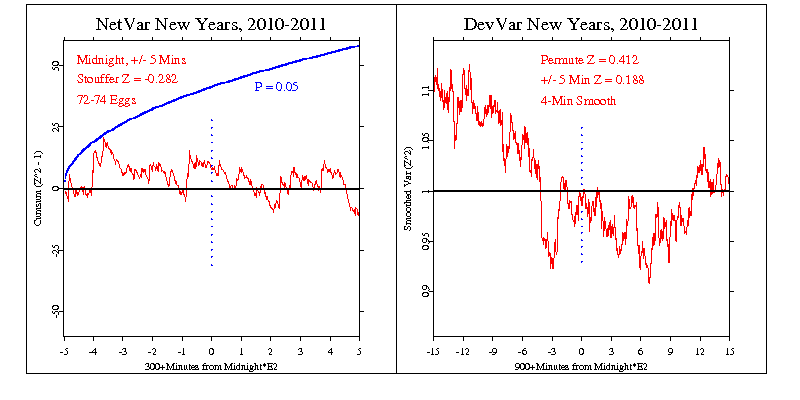# New Year's 2010-2011

This year again we use the longstanding predictions of a meanshift (squared Stouffer Z) during 10 minutes surrounding midnight, and a variance decrease with a minimum at midnight. These two measures have been assessed for each New Year since 1998-1999, a total of 13 years.

The probability for the squared Stouffer Z for this year is not significant at p=0.611 with Z=-0.282

For the Variance decrease, a comparison with the distribution of 10000 permutations of the data indicates a modest probability of 0.340 and Z=0.412, in accord with the prediction.In addition to the two figures above representing the results for the current year, a composite over the 13 years is shown for the variance decrease prediction, which is supported with a p-value of 0.030 and Z=1.879. The meanshift is slightly positive, with Z = 0.21.

The following figure is a graph of the average of the variance over 12-years.